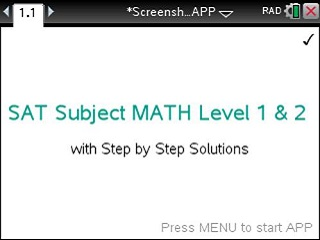# SAT Subject Test Math Level 1 & 2

## App Purchase

Enter the last 8 digits of your 27-digit TI-Nspire's Product ID.

Located under 5:Settings → 4:Status → About ID may look like: 1008000007206E210B0BD92F455 HELP. If this was your ID you would only type in BD92F455.

At the end of the PayPal checkout, you will be sent an email containing your app. Read Installation InstructionsPrice:\$49.95

## Description

https://youtu.be/KEKCubX_FXw
• The most comprehensive SAT Math Subject Test APP for calculators.
• Users have boosted their SAT MATH knowledge & scores
• Easy to use. Just plug in the equation or numbers and the correct answer shows.
• Test the FREE Trial here.

## FUNCTIONALITY & MENU ITEMS OF APP :

### Algebra

• Solve any Equation or Inequality
• Solve 2x2 system - Step by Step
• Read: PEMDAS - Order of Operation
• Simplify & Evaluate
• Factor
• Powers
• Expand/Distribute
• Find GCD & LCM
• Find Common Denominator
• Find Proper Fractions
• Solve Proportion (Ratio) Problems
• Absolute and Percent Change

### Functions

• All-in-one-Function Explorer
• Evaluate Function
• Intersection of 2 Functions
• Find f+g
• Composition of 2 Functions f(g(x))
• Find [f(x+h)-f(x)]/h
• Find Inverse function

### Points and Lines

• Find Slope
• Find y=mx+b
• Find Point Slope & y=mx+b given Pt & Slope
• Check if 2 Lines are Parallel or Perpendicular
• Find Parallel and Perpendicular Lines
• Read: Absolute Value Function |x|

### Quadratic Equations and Complex Numbers

• Complete the Square
• Complete the Square to find Zeros
• Complete the Square to find Vertex
• One Complex Number : All-in-one-Explorer
• Two Complex Numbers : All-in-one-Explorer

### Polynomials

• All-in-one-Polynomial Explorer
• Find Degree

### Exponents and Logarithms

• Solve any Equation
• Solve using the Rule 72
• Solve Money/Exponential Growth Problems
• Evaluate Logarithms
• Logarithm Solver
• Change of Base

### Sequences & Series

• Explicit Sequence & Partial Sum
• Recursive Sequence & Partial Sum
• Sequence Formula Finder
• Geometric Sequence & Series
• Arithmetic Sequence

### Matrices

• All in one Matrix A Explorer
• Two Matrices: A+B
• Find the Inverse of A
• Find the Determinant of A
• Row Echolon(A)
• Reverse Row Echolon(A) - STEPS
• Solve System of Equations A*X=B
• Cramer Rule to solve A*X=B

### Trigonometry

• Solve any 90 degree Triangle
• Solve any triangle
• SSS-Case
• SAS-Case
• SSA-Case
• SAA-Case
• Evaluate sin(x)

### Circles

• Read: Unit Circle: Angles & Coordinates
• Read: Circle Properties & Formulas
• Find Sector
• Arc Length Solver

### Geometry

• 2D: Circle Solver
• 2D: Find Sector
• 2D: Arc Length Solver
• 2D: Pythagorean Theorem
• 2D: Triangle Solver
• 2D: Square Solver
• 2D: Rectangle Solver
• 2D: Parallelogram Solver
• 2D: Rhombus Solver
• 2D: Trapezoid Solver
• 3D: Sphere Solver
• 3D: Cube Solver
• 3D: Cylinder Solver
• 3D: Cone Solver
• 3D: Rectangular Prism Solver

### Convert

• Polar Coordinates <-> (x,y)
• Degree to Degree/Minutes/Seconds